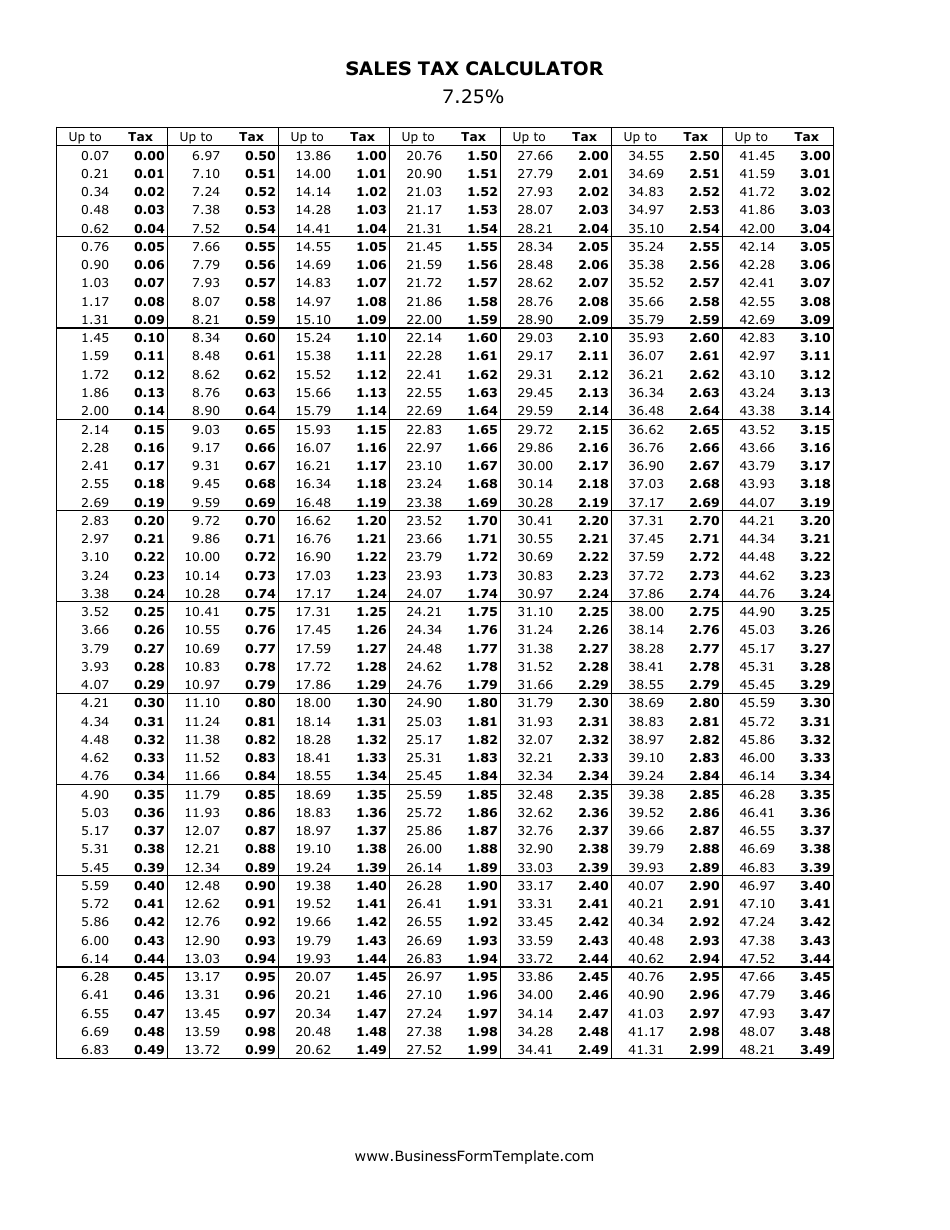# 7.25% Sales Tax Calculator

The 7.25% Sales Tax Calculator is used to calculate the amount of sales tax you would have to pay on a purchase. It helps determine the total cost of an item after adding the sales tax.

The 7.25% Sales Tax Calculator is typically filed by the business or merchant collecting the sales tax from customers.

## FAQ

Q: How do I calculate sales tax?
A: To calculate sales tax, multiply the purchase amount by the sales tax rate.

Q: What is the sales tax rate?
A: The sales tax rate is 7.25%.

Q: How much is the sales tax on a \$50 purchase?
A: The sales tax on a \$50 purchase is \$3.63.

Q: What is the total amount including sales tax on a \$100 purchase?
A: The total amount including sales tax on a \$100 purchase is \$107.25.

Q: How much is the sales tax on a \$200 purchase?
A: The sales tax on a \$200 purchase is \$14.50.

Q: How can I calculate the total amount including sales tax?
A: To calculate the total amount including sales tax, add the purchase amount and the sales tax amount.

•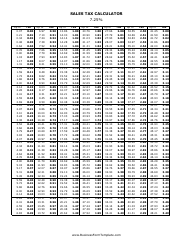1

•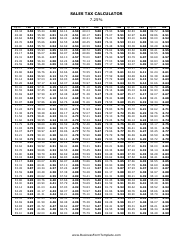2

•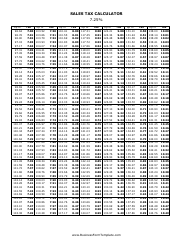3

•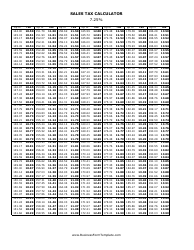4

•